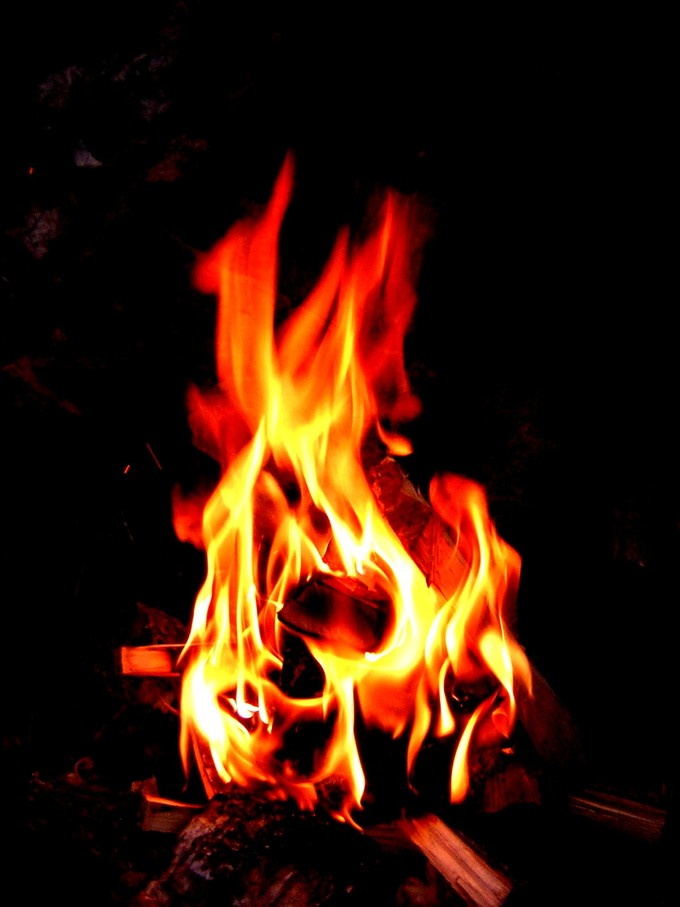## Combustion Analysis

#### Learning Objective

• Describe the process of combustion analysis.

#### Key Points

• Combustion is the process of burning an organic compound in oxygen to produce energy, carbon dioxide, and water vapor.
• In combustion analysis, a sample of known mass is combusted, and the resulting carbon dioxide and water vapor are captured and weighed.
• The relative amounts of carbon, hydrogen, and oxygen in the starting compound can be determined from the masses of the products of the combustion reaction.
• Combustion analysis can thus be used to determine the empirical formula of an unknown organic compound.

#### Terms

• combustion analysisUsing burning to determine the elemental composition of an organic compound. The compound is burned, the products are collected and weighed, and the composition is determined.
• combustionA process wherein a fuel is combined with oxygen, usually at high temperature, releasing heat, carbon dioxide and water vapor.

Combustion analysis is an elemental analytical technique used on solid and liquid organic compounds. It can determine the relative amounts of carbon, hydrogen, oxygen in compounds, and occasionally can also identify the amounts nitrogen and sulfur in compounds. This technique was invented by Joseph Louis Gay-Lussac.

## Combustion

Combustion analysis is commonly used to analyze samples of unknown chemical formula. It requires only milligrams of a sample. The sample is weighed and then fully combusted at a high temperature in the presence of excess oxygen, which produces carbon dioxide and water.

One example of a simple combustion reaction is the combustion of methane:

$CH_{4} + 2\:O_{2} \rightarrow CO_{2} + 2\:H_{2}O + energy$

Another common example of combustion is the burning of wood to produce thermal energy. When 1 mole of propane (C3H8) is burned in excess oxygen, 3 moles of CO2 and 4 moles of H2O are produced.CombustionEnergy is released in the form of flames as the fuel combusts.

## Combustion Analysis

In combustion analysis, the products, carbon dioxide and water vapor, are trapped by absorption onto reactive solids located in tubes above the reaction vessel. These tubes can then be weighed to determine the absorbed masses of carbon dioxide and water.

• The mass of carbon in the starting material is determined by a 1:1 ratio with the mass of carbon dioxide produced (as in the combustion reaction for methane already displayed).
• The initial hydrogen mass is determined by a 2:1 ratio with the amount of water produced.

The data and the ratios can then be used to calculate the empirical formula of the unknown sample. Combustion analysis can also be performed using a CHN analyzer, which uses gas chromatography to analyze the combustion products.